## Tuesday, December 21, 2010

### Visualizing 7 dimensional simplex

Suppose we'd like to visualize a set of joint probabilities realizable by distributions of 3 binary variables.

It is a 7 dimensional regular simplex, and we could draw a lower dimensional section of it. There's an infinite number of sections, but there aren't that many *interesting* ones.

As an example, suppose we want to find a good 2 dimensional section of a 3 dimensional regular simplex. It seems reasonable to restrict attention to sections that go through simplex center and two other points, each of which is a vertex or a center of some edge or face. There are just 2 such sections determined up to rotation/reflection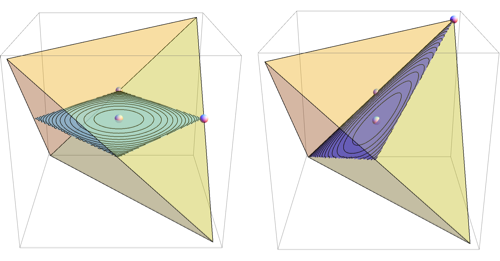For 7 dimensional simplex, we could similarly take 3 dimensional sections determined by simplex center and 3 points, each a centroid of some set of vertices. This gives 49 distinct shapes, shown below.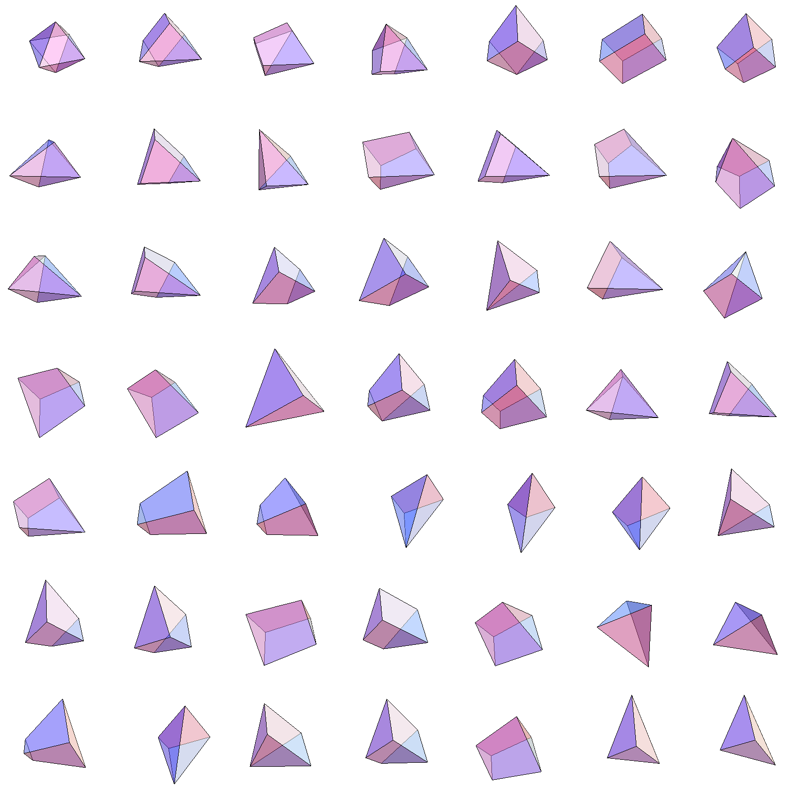Among those, there's just one section that intersects all 8 faces of the simplex and preserves the underlying symmetry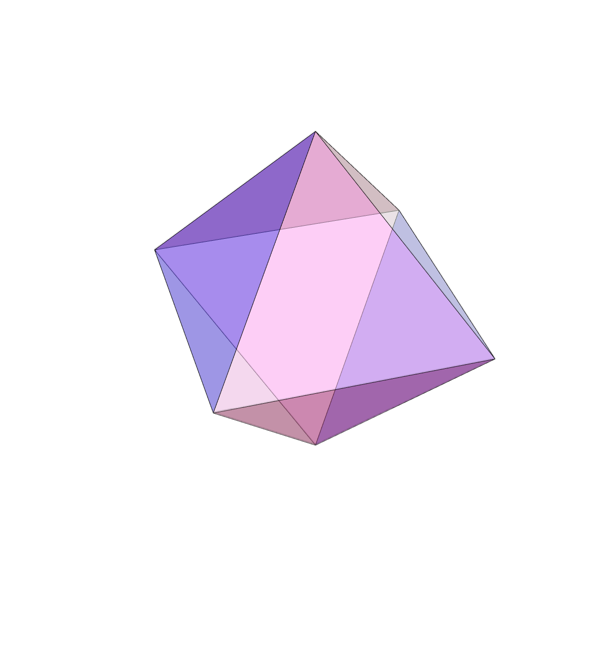Also known as the regular octahedron.

Implementation details

## Wednesday, December 15, 2010

### Computationally nice structures

One approach to solving hard problems is to break them into computationally efficient parts.

For instance, Globerson/Jaakkola do approximate counting by decomposing graph into planar graphs. In each part, the problem reduces to perfect matchings which can be solved efficiently on planar graph. Bouchet/Jordan decompose counting bipartite perfect matchings into much easier problems that count matchings perfect on "one side" of the graph. Sontag/Jaakola solve subproblems on star-shaped subgraphs of original graph, the symmetry of star graph enabling a very fast solution to each subproblem.

I recently came across graph class database which gives information on thousands of graph classes. It shows dozens (hundreds?) distinct tractable classes for exact maximum independent set, and most of them seem to have unbounded tree width. I haven't heard of most of those classes and would be curious to know how many distinctly interesting algorithms they correspond to

Here are a few computationally nice structures I've seen employed in Machine Learning and Vision literature

### Upper-bounded tree width

Everything is easy via tree decomposition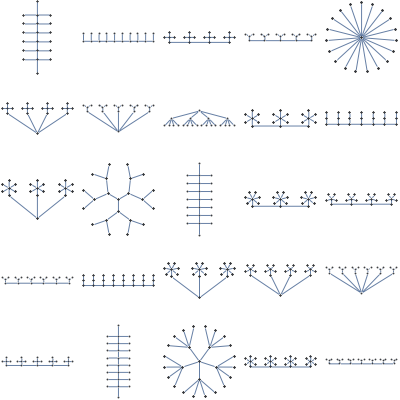### Lower-bounded girth

Almost everything is easy via loopy belief propagation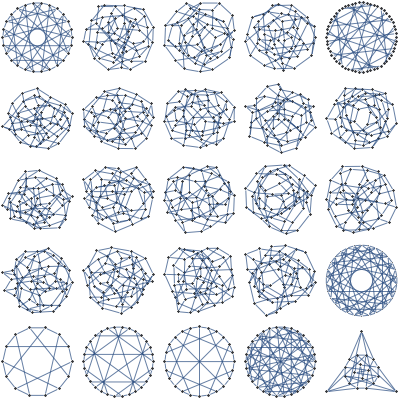### Planar

Easy minimization of submodular functions. Easy counting of certain structures by reduction to perfect matchings.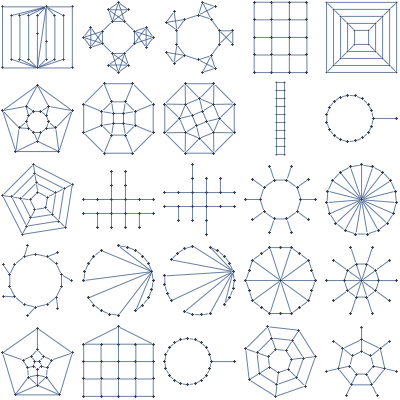### Complete

Easy counting of unweighted local structures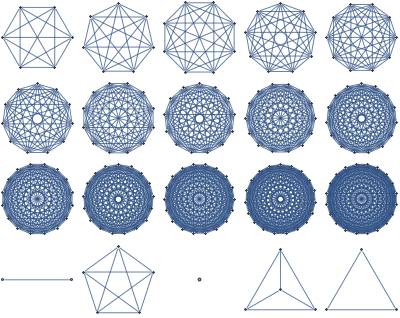### Perfect

Easy MAP inference with NAND potentials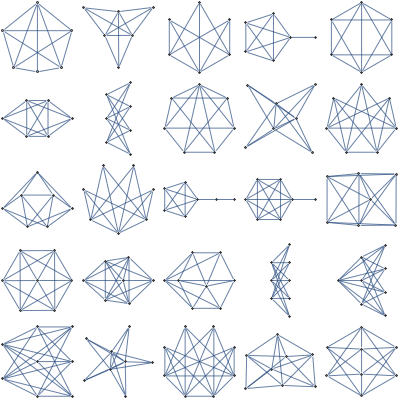### Star

Very efficient updates for MAP inference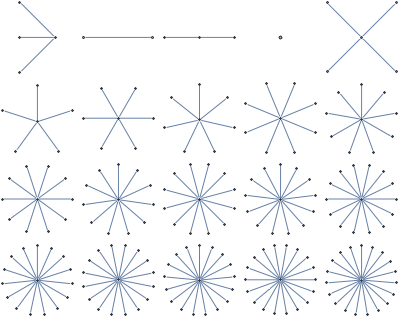### Claw-free

A generalization of line graphs. Easy maximum independent set, and counting by reduction to matchings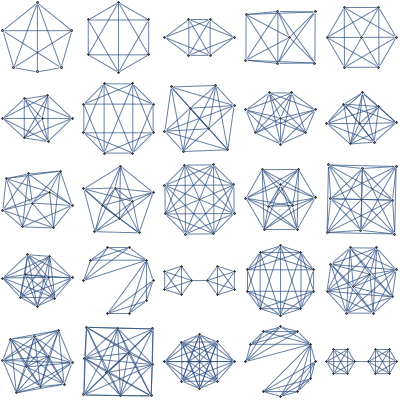Any other useful classes I missed?
Mathematica notebook

## Sunday, December 12, 2010

### NIPS 2010 highlights

A few "connection to other fields" papers I found interesting

• Variational Inference over Combinatorial Spaces. Alexandre Bouchard-Cote, Michael Jordan:
Extend variational formulation to upper bound the number of combinatorial structures with global constraints, like perfect matchings and TSP tours. The trick is to represent the space of structures as an intersection of spaces that are tractable to count. For perfect matchings, it is tractable to count edge sets that satisfy perfect matching constraint on one side, so you can represent the total space as intersection of perfect matchings that are "left-legal" and "right-legal". In experiments, the algorithm gave same accuracy for perfect matchings as existing PTAS based on Jerrum's technique, but 10 times faster.

• Learning Efficient Markov Networks. Vibhav Gogate, William Webb, Pedro Domingos:
Learn high tree-width models and ensure efficient inference by restricting potential functions to a compact representation as a tree of AND/OR predicates. The problem of learning is reduced to symmetric submodular function minimization which can be done in $O(n^3)$ time with Queyranne's algorithm. For experiments, $O(n^3)$ was too slow, but they give a heuristic which worked to give state-of-the-art results in a practical dataset.

• MAP estimation in Binary MRFs via Bipartite Multi-cuts. Sashank Jakkam Reddi, Sunita Sarawagi, Sundar Vishwanathan:
Reduces MAP inference in binary Markov Fields to multi-commodity flow problem

• Worst-case bounds on the quality of max-product fixed-points. Meritxell Vinyals, Jesús Cerquides, Alessandro Farinelli, Juan Antonio Rodríguez-Aguilar:
Uses an inequality recently obtained in multi-agent literature to bound difference between true max-marginal and max-product max-marginal in terms of graph structure

Some interesting applied papers

• Segmentation as maximum weight independent set. William Brendel, Sinisa Todorovic:
You want to segment your image into regions, but the initial candidate regions are overlapping fragments. Real segmentations don't have overlaps, turn this into MWIS problem to find good solution satisfying these mutual exclusivity constraints

• Efficient Minimization of Decomposable Submodular Functions. Peter Stobbe, Andreas Krause:
Applies some recent techniques from non-smooth optimization to Lovasz extension of submodular function

• Optimal Web-Scale Tiering as a Flow Problem. Gilbert Leung, Novi Quadrianto, Alexander Smola, Kostas Tsioutsiouliklis:
Linear integer programming to optimize cache arrangement for 84 million web-pages on Yahoo servers

At least four groups (Bach, Mosci, Jia, Micchelli) had papers on "structured sparsity". Traditional approach to sparse learning is to maximize the number of 0's in the parameter vector. The new idea is to incorporate knowledge on the pattern of 0 values.

Andy Mueller has some more highlights

## Sunday, December 05, 2010

### Mathematica blog

Most graphics and formulas that appear in this blog were created with help of Mathematica. I'll keep a separate blog with Mathematica tips.

## Friday, December 03, 2010

### Moving away from traditional peer-review

Common complaint about current publishing model is that sometimes good papers get rejected. A striking example is that David Lowe's SIFT algorithm was rejected multiple times from vision venues. The author then assumed that vision community is not interested, and applied for patent intended promote it just for industrial applications. As a result, what's arguably the most popular key-point detection algorithm is not free to use. This may also say something about limitation of our patent system.

You can see a few more examples in the inaugural issue of Mathematica Rejecta.

Yann LeCun proposes a solution to the problem.
His idea is like non-anonymous stack overflow, where you could search for papers that got several positive reviews, or ones that were positively reviewed by a specific reviewer.

I think the problem is not as serious now as it was before the Internet. Lobachevsky's work was rejected from peer-reviewed journals and might have faced obscurity if not for personal intervention of Gauss.

Today, if your result is obviously important, putting it up on your webpage may end up giving you as much publicity as a publication in a top journal, Ryan Williams latest CS-theoretical result is an example. This approach can also work to screen for errors, as we have seen in the case of Vinay Deolalikar P!=NP paper.

University of Minnesota officially looked into ways of assessing "new forms of scholarship in consideration of academic promotion. Andrew Gelman notices that his most influential papers were published in low ranking journals.

The bottom line is that world is shifting away from the traditional peer-reviewed publication model. Good results don't always need an official stamp of approval to be recognized, and perhaps in 30 years, mentioning the journals where you published at an academic interview will be as bad as coming to a software engineer interview with a heap of Certificates of Proficiency

This reminds me of a faculty candidate that came to interview to our Computer Science department once. He gave a good presentation but didn't get the job. I asked the person in charge of the hiring committee why, the answer was -- "he had too many publications, they couldn't all be good"

## Tuesday, November 30, 2010

### Visualizing Tree Decompositions

In order to do exact probabilistic inference on real life network efficiently, one must find a good Tree Decomposition of the network. This process is known as the Junction Tree algorithm. It's a bit hard to visualize the result, but while browsing tree decomposition graph pages on Wikipedia, I got an idea. Instead of bags with variables, we plot it as a collection of colored strips where each strip corresponds to a variable and Running Intersection property guarantees there will be no breaks.

Here's the result for the width-7 tree decomposition of the moralized Barley network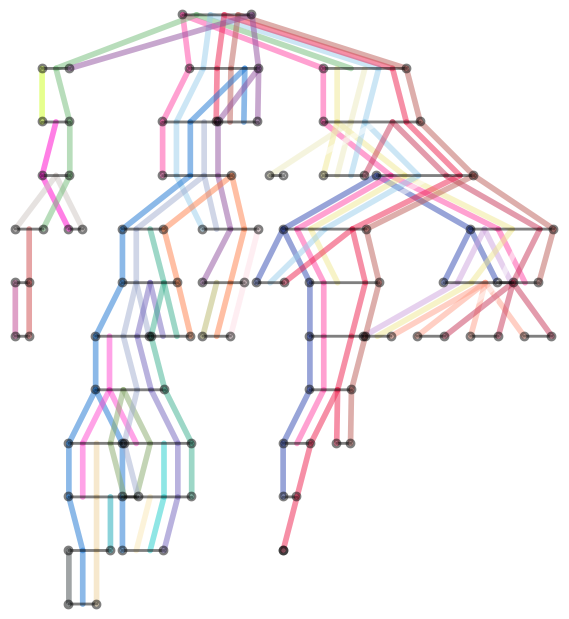Mathematica source

## Sunday, November 28, 2010

### Springer temporarily opens "Machine Learning" journal

If you never heard of the journal "Machine Learning", it used to be number 1 ranked journal in ML, until board of editors resigned and founded the Journal of Machine Learning Research, possibly the greatest success story in open-access publishing

## Friday, November 19, 2010

### Prediction competitions

I just came across kaggle.com which is a platform for "s a platform for data prediction competitions." From brief glance, it seems the goal is to automate Netflix-prize-like competitions. It seems four competitions are currently active

## Wednesday, November 17, 2010

### SVM plots

Ulrich Bodenhofer has made some nifty SVM visualization code. One is Mathematica notebooks that takes libSVM model files and visualizes them, another one generates plots of Bayes optimal classifier on some synthetic 2d problems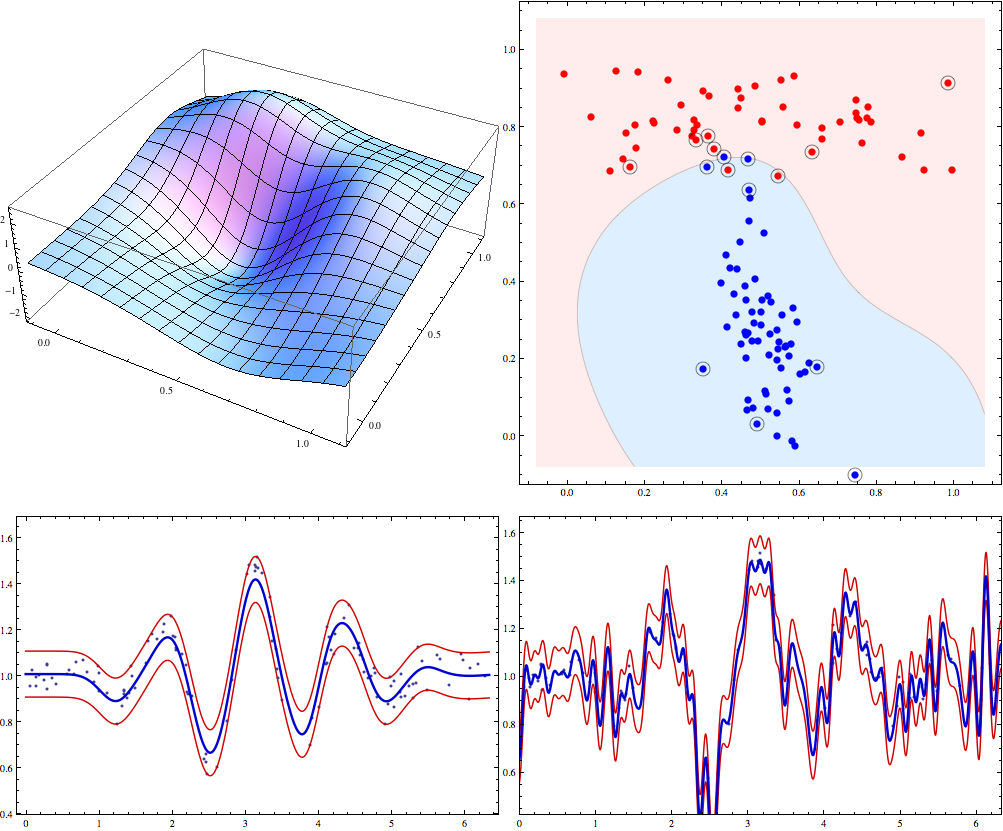## Tuesday, November 02, 2010

### Importance of naming things

Searching for "NIPS" in google blog-search mainly produces posts about nipples, my "CRF" subscription on delicious recently flooded me with links about "Chronic Renal Failure" (apparently a common feline ailment), and you can guess what I got when trying to find Bill Sutherlands "Beautiful Models" book (it's about statistical physics models).

Other unfortunate name choices -- hard-ass (hard as satisfiability, proposed for NP-complete problems before they had a name), Go programming language, hardcore model, Michael Jordan

As a rule of thumb, when coming up with a name for new technique, conference, concept, etc, it should satisfy at least one of two criteria:

• be funny (ie RODEO ("regularization of derivative expectation operator") as an improvement on the LASSO (least absolute shrinkage and selection operator))

## Thursday, October 28, 2010

### Sometimes simplest learners are best -- WinnowTag.com experiment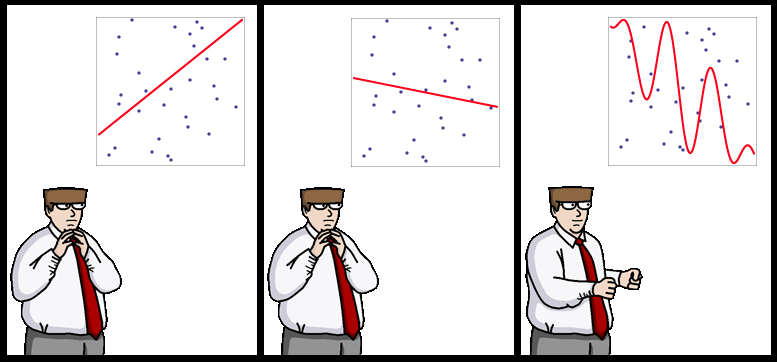In 1993, R.Holte noted that "Very simple classification rules perform well on most commonly used datasets". His very simple rules are basically binary classifiers that make decision based on value of a single attribute.

In the race for latest and greatest classifiers it's easy to forget that many kinds of classification tasks in 1993 still come up today, so conclusions of Holte's paper still apply.

I came across this effect couple of years ago on a set of document categorization experiments. Folks at WinnowTag wanted me to look into various methods for automatically tagging news/blog items. The idea was that a user manually tags some articles, the system learns and gradually takes over the role of tagging. You can approach it as a straight-forward binary classification task since number of errors represents the number of tag additions/deletions needed from user to fix the tagging.

At first I thought about jumping in and coding some latest and greatest approach for multi-tag labeling like one in from NIPS'04 paper, but as a sanity check, I decided to try existing Weka's classifiers on the dataset.

Nice thing about Weka is that it provides a unified command line interface to all of its classifiers, so you could make a simple Python script like this to run 64 different classifiers on your classification task with default parameters provided by Weka.

4000 hours of EC2 runs later, I got the results. Simplest classifiers like Naive Bayes, OneR (from Holt's paper) made the smallest number of errors. Weka's BayesNet, J48, VotedPerceptron, LibSVM performed as bad or worse as predicting "False" for every tag. Logistic Regression, Neural Networks, AdaboostM1 failed to finish after a day of training.

In retrospect, it makes sense -- in the original dataset there were 35 tags and 5-40 documents per tag, sometimes as small as a couple of sentences, and with the amount of inherent noise in tagging, that's simply not enough data to identify some of the deeper patterns that higher complexity classifiers are made for. In this settings, I wouldn't expect "advanced" classifiers to give a significant improvement over Naive Bayes even after significant tuning of hyper-parameters

It's been suggested that more data beats better algorithms, but here, I would say that when you have little data/prior knowledge, simplest learners work as well as the most advanced ones.

PS: $1 for the caption to the strip above ## Tuesday, October 26, 2010 ### Times they are a'changin' Suresh points out that at this year's FOCS, not a single person wanted printed proceedings, whereas few years ago, a third of the audience would ask for printed version. I see a similar shift happening with technical books. Purists say that you can't beat the convenience of browsing a real book, but I say that you can't beat the convenience of having access to all your books wherever you go. In the last 6 years, I came across about a hundred technical books I considered interesting enough to get an electronic version of, and since publishers didn't provide electronic versions, this often meant me going to the library and scanning the book myself. One of my first blog posts was on the subject of book scanning. Today, almost 6 years later, I almost never need to use the scanner, because almost every book I need is already available in electronic form, scanned by someone and circulating freely on the Internet. Increasing number of those illegally circulating books are not even scans, but look like bona fide original electronic versions. Number of legally available electronic versions is also increasing, recent examples I came across are Boyd's Convex Optimization, McCallaugh's Tensor Methods in Statistics and Szeliski's Computer Vision: Algorithms and Applications. Times they are a'changin' ! ## Friday, October 22, 2010 ### ICML topic trends David Mimno fit a Dirichlet-multinomial to ICML papers 2004-2008. Seems like "real world" problems are going strong, while boosting took a serious dive ## Wednesday, October 13, 2010 ### Why do we need integrals in Computer Science? A comment on previous post asked why we need integrals for computer science. One reason is that combinatorial expressions often have representation in terms of integrals. Consider binomial coefficients. We have the following $${n \choose \frac{n+d}{2}}=\frac{1}{2 \pi} \int_{-\pi}^\pi e^{-\mathbb{i} q d} (2\cos q)^n dq$$ To see where this comes from, consider that$2 \cos x=e^{-\mathbb{i}\pi}+e^{\mathbb{i}\pi}$is the generating function for number of one step walks starting at 0. Coefficients give number of those walks ending at 1 and -1. Raise it to$n$and apply inverse Fourier transform to get number of$n$step walks ending at at$d$, which has an alternative representation in terms of binomial coefficient. Here's what the result looks like for arbitrary$d$with$n=10$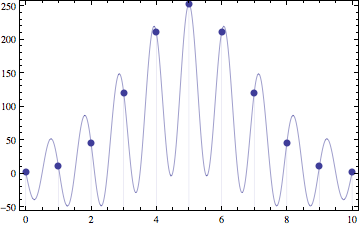Another way to get binomial coefficients is to note that they form coefficients of power of$x$in expansion of$(1+x)^n$. Use Cauchy's integral formula to recover the coefficient. We get the following $${n \choose k} = \frac{1}{2\pi \mathbb{i}}\oint_c \frac{(1+x)^n}{x^{k+1}}$$ Contour integral is counter-clockwise around some contour that encircles the origin. For instance, you can use the following contour.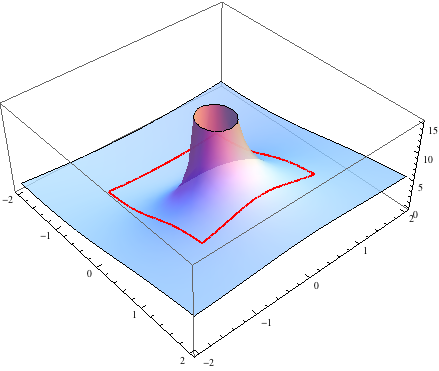Mathematica notebook ## Sunday, October 03, 2010 ### Theoretical CS cheat sheet Thanks to John Cook for pointing it out ## Saturday, October 02, 2010 ### Universal Laws and Computational Irreducibility • Terry Tao's article on universality. • Stephen Wolfram's speech on future special functions. They give opposing perspective -- in the end of speech Stephen Wolfram argues that most processes in universe are "computationally irreducible" and we have no hope to simulating them accurately, whereas Terry Tao's article gives examples of many things in real world obeying a small set of simple laws ## Saturday, September 25, 2010 ### Order Matters Suppose I have an invertible function$f(x)$. In a perfect world, the following holds $$x=f(f^{-1}(x))=f^{-1}(f(x))$$ To see what happens in a real world, consider the following $$D=\left(\begin{matrix}1&1 \\\\ 1&0\end{matrix}\right)^{\otimes\ d}$$ $$f(\mathbf{x})=D\exp (\mathbf{x} D)$$$f(x)$maps natural parameters$x$to mean value parameters$\mu$in an exponential family over$\{0,1\}^d$Let$d=6, x=<1/2,1/2,1/2,\ldots,1/2,1>$. Using machine arithmetic you get the following $$\|f^{-1}(f(x))-x\|_{\infty}\approx 2.6\times 10^{-15}$$ and $$\|f(f^{-1}(x))-x\|_{\infty} \approx 0.21$$ ## Friday, September 24, 2010 ### Updated Machine Learning/Statistics blog list I recently raked the blogosphere for interesting new Machine Learning/math blogs and got a high recall, low precision list of 87, here. ## Thursday, September 16, 2010 ### Dirac integration trick Suppose X is distributed as n-dimensional Gaussian with 0 mean and concentration matrix$A$and you need conditional distribution of$P(\mathbf{x}|\mathbf{vx}=\mathbf{0})$where$\mathbf{v}$is some unit norm vector. To normalize this density you need to integrate$\exp(-\mathbf{x}'A\mathbf{x})$over subspace of$\mathbb{R}^n$orthogonal to$\mathbf{v}$, how do you do it? Take the Dirac delta function. A nice property is that $$\int dx \delta(x) f(x) = f(0)$$ Write it in terms of Fourier transform $$\delta(x)=\int dk\exp(-2\pi i k x)$$ Now we can integrate our Gaussian over whole domain, but multiply by$\delta(\mathbf{v}' \mathbf{x})$to set to 0 density in regions not orthogonal to$\mathbf{v}$. Changing integration order we get $$Z_v=\int dk \int d\mathbf{x} \exp(-\mathbf{x}'A\mathbf{x}-2\pi i k \mathbf {v}' \mathbf{x})$$ Second integral is a standard Gaussian integral and can be solved by completing the square (Appendix B, Bishop's "Neural Networks"). The first then becomes another Gaussian integral, with final result $$Z_v=\left(\frac{\pi^{d-1}} {|A| \mathbf{v'}A^{-1}\mathbf{v}}\right)^\frac{1}{2}$$ ## Sunday, September 05, 2010 ### MaxEnt or Bayesian? Foundations of probabilistic inference is often a subject of much disagreement, with some leading Bayesian sometimes going as far as to say that MaxEnt method doesn't make any sense, and the MaxEnt camp picking on the issue of subjectivity. The way I see it, MaxEnt and Bayesian approaches are just different ways of using external information to pick a probability distribution. With Bayesian approach, you are given data and a prior. Bayesian inference then comes out as a natural extension of logic to probabilities. See Ch.1 of Cox's Algebra of Probable Inference for full axiomatic derivation. With MaxEnt approach, you are given a set of valid probability distributions. You can then justify MaxEnt axiomatically but personally I like the motivation given in Jaynes book, Probability Theory: The Logic of Science (in particular Ch.11). Roughly speaking, it goes as follows. Suppose we have a distibution$p$over$d$outcomes. We say that a sequence of$n$observations is explained by distribution$p$if relative counts of outcomes in this sequence match$p$exactly. The number of sequences matched by$p$is the following quantity $$c(p)=\frac{n!}{(np_1)!(np_2)!\cdots (np_d)!}$$ If we have a set of valid distributions$p$, it then makes sense to pick the one with highest$c(p)$because it'll fit the greatest number of possible future datasets. Approximating$n!$by$n^n$we get the following $$\frac{1}{n}\log c(p)\approx \frac{1}{n}\log \frac{n^n}{(np_1)^{np_1}(np_2)^{np_2}\cdots (np_d)^{np_d}}=H(p)$$ Hence picking distribution with highest H(p) usually means picking the distribution that will be able to explain the most possible observation sequences. Because it's an approximation, there can be exceptions, for instance distributions$(\frac{12}{18},\frac{3}{18},\frac{3}{18})$and$(\frac{9}{18},\frac{8}{18},\frac{1}{18})\$ have equal entropy, but the latter explains 18% more length 18 sequences.

To carry out the inference, Bayesian approach needs to start with a prior, whereas MaxEnt approach needs to start with a set of valid distributions. In practice people often use constant function for prior, and "distributions for which expected feature counts match observed feature counts" for the valid set of distributions.

Note that when dealing with continuous distributions, result of MaxEnt inference depends on the choice of measure over our set of distributions, so I think it's hard to justify in continuous case.

Here are some papers I scanned a while back on foundations of Bayesian and MaxEnt approaches.

## Saturday, September 04, 2010

### non-asymptotic uses of Central Limit Theorem

Suppose we throw a fair coin n times and estimate it's bias by averaging the number of heads observed. What is the squared error of this estimator?

Using standard binomial identities we can calculate this quantity exactly

$$\sum_{k=0}^n {n\choose k} 2^{-n} (\frac{k}{n}-\frac{1}{2})^2=\frac{1}{4n}$$

Another approach is to use the Central Limit Theorem to approximate exact density with a Gaussian. Using differentiation trick to evaluate the Gaussian integral we get the following estimate of the error

$$\int_{-\infty}^\infty \sqrt{\frac{2}{\pi n}} \exp(-2n(\frac{k}{n}-\frac{1}{2})^2)(\frac{k}{n}-\frac{1}{2})^2 dk=\frac{1}{4n}$$

You can see that using "large-n" approximation in place of exact density gives us the exact result for the error! This hints that Central Limit Theorem could be good for more than just asymptotics.

To see why this approximation gives exact result, rearrange the densities above to get an approximate value of k'th binomial coefficient

$${n\choose k} \approx \sqrt{\frac{2}{\pi n}} \exp(-2n(\frac{k}{n}-\frac{1}{2})^2) 2^n$$

Below are exact and approximate binomial coefficients for n=10, you can see it's fairly close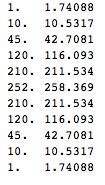This is similar to an approximation we'd get if we used Stirling's approximation. However, Stirling's approximation always overestimates the coefficient, whereas error of this approximation is more evenly distributed. Here's plot of logarithm of error of our approximation and one using Stirling's, top curve is Stirling's approximation.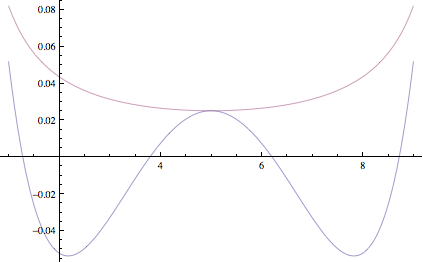You can see that errors in the estimate due to CLT are sometimes negative and sometimes positive and in the variance calculation above, they happen to cancel out exactly

## Monday, August 30, 2010

### New Machine Learning blog

By Frank Nielsen, with focus on information geometry, http://blog.informationgeometry.org/

## Sunday, August 15, 2010

### Method of Types

After following some discussions on overflow sites, I re-read Shannon/Cover's coverage of method of types, and want to summarize it here because of how useful and underappreciated it is.

A type or type class is basically an empirical distribution for a sequence of n independent events. For instance, suppose we flip coin n=2 times. We have 3 type classes, all heads, all tails, one head/one tail. Size of the type class is the number of sequences in that class. Here, two of our classes have size 1 and "one head/one tail" has size 2.

Since the number of types grows polynomially with n, while number of observation sequences grows exponentially, some type classes end up getting an exponential number of sequences. Since constants of exponential growth are different for different types, probability mass ends up getting concentrated in a small number of types and several important properties follow from this concentration behavior.

In particular, Thomas/Cover use method of types to formalize and prove the following statements in Chapter 12 of "Information Theory and Statistics" (1991).
• Probability of hypothesis E under distribution P is determined by the probability of closest member of E to P (Sanov's theorem)
• To test whether data x was generated by p or q, it's sufficient to only look at the ratio of probabilities of x under p and under q (Neyman-Pearson lemma)
• When data is generated by p or q and you are trying to tell which one it is from data, probability of making a mistake for an optimal classifier is bounded by exp(-n max(KL(p,q),KL(q,p)). A tighter bound is to use KL(x,p)=KL(x,q) where x is the "halfway point" between distributions (Chernoff's theorem)
The last point actually gives an alternative (more intuitive IMHO) motivation for Fisher's information metric. It's known that when w1 and w2 are close, KL-divergence between parametrized distributions p(w1) and p(w2) behaves as the square of Mahalonobis distance between w1 and w2 with Fisher Information Matrix as the covariance matrix. So closeness in Fisher Information Metric now corresponds to difficulty of telling two distributions apart based on data. This notion can be used to define the volume of a probability distribution family by counting "balls" of indistinguishable probability densities. It seems like a very "aesthetically" appealing approach to model selection, although I haven't seen it used much in practice, perhaps because so few people in machine learning know differential geometry.

Rodriguez says it outperforms BIC and AIC by orders of magnitude

Resources

## Tuesday, August 10, 2010

### Interesting Stack Exchanges

As I discovered recently, stack exchanges can be pretty fun, informative (and time consuming!) way to discuss issues related to machine learning. Here are some relevant ones I found

There's also stack exchange for Machine Learning and Computer Vision that are in proposal phase. Any interesting ones I missed?

Machine Learning stack exchange is right now undergoing definition. Please go and vote on interesting questions to help define it as a research-level discussion forum